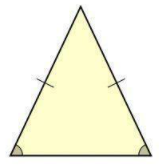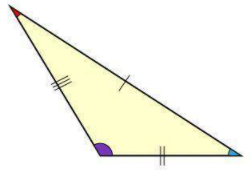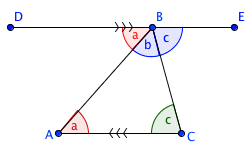# Properties of Triangles

There are three types of triangles based on how many sides or angles that are equal. Those are, the Equilateral Triangle with all three sides equal, and all angles equal (always 60°), the Isosceles Triangle with all two sides equal and two angles equal, and lastly, the Scalene Triangle with none of the sides equal and none of the angles equal.### Equilateral Triangle### Isosceles Triangle### Scalene Triangle

Once we classify the triangle, we can use this information to solve for missing sides/angles.

## Sum of Interior Angles

In a triangle, the three interior angles always add to $$180°$$:

$$\angle A + \angle B + \angle C = 180°$$

Refer to the diagram below. A triangle with angles $$\angle A,\ \angle B$$ and $$\angle C$$ is extended with a parallel line $$\vec{DE}$$, such that $$\vec{AC}$$ is parallel to $$\vec{DE}$$. From this we can use a "Z" pattern rule to see that the three angles make a straight line around point $$B$$. We know that a straight line is $$180°$$ so the sum of the interior angles of the triangle must also be $$180°$$!An equilateral triangle has side lengths $$a=3$$ and $$b=3$$. Calculate the missing length.

In a scalene triangle, one angle is $$116°$$, another is $$35°$$. What's the degree of the third angle?"

If the height of an equilateral triangle is $$2\sqrt{3}$$. Calculate the side length.